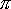QUESTION #328

# What is the easiest way to calculate the earth's athmoshpere's weight?

Since we're estimating, use 4000 miles for the Earth's radius, and calculate the area of its surface with the formula for the area of a sphere: 4 xx (radius)2.